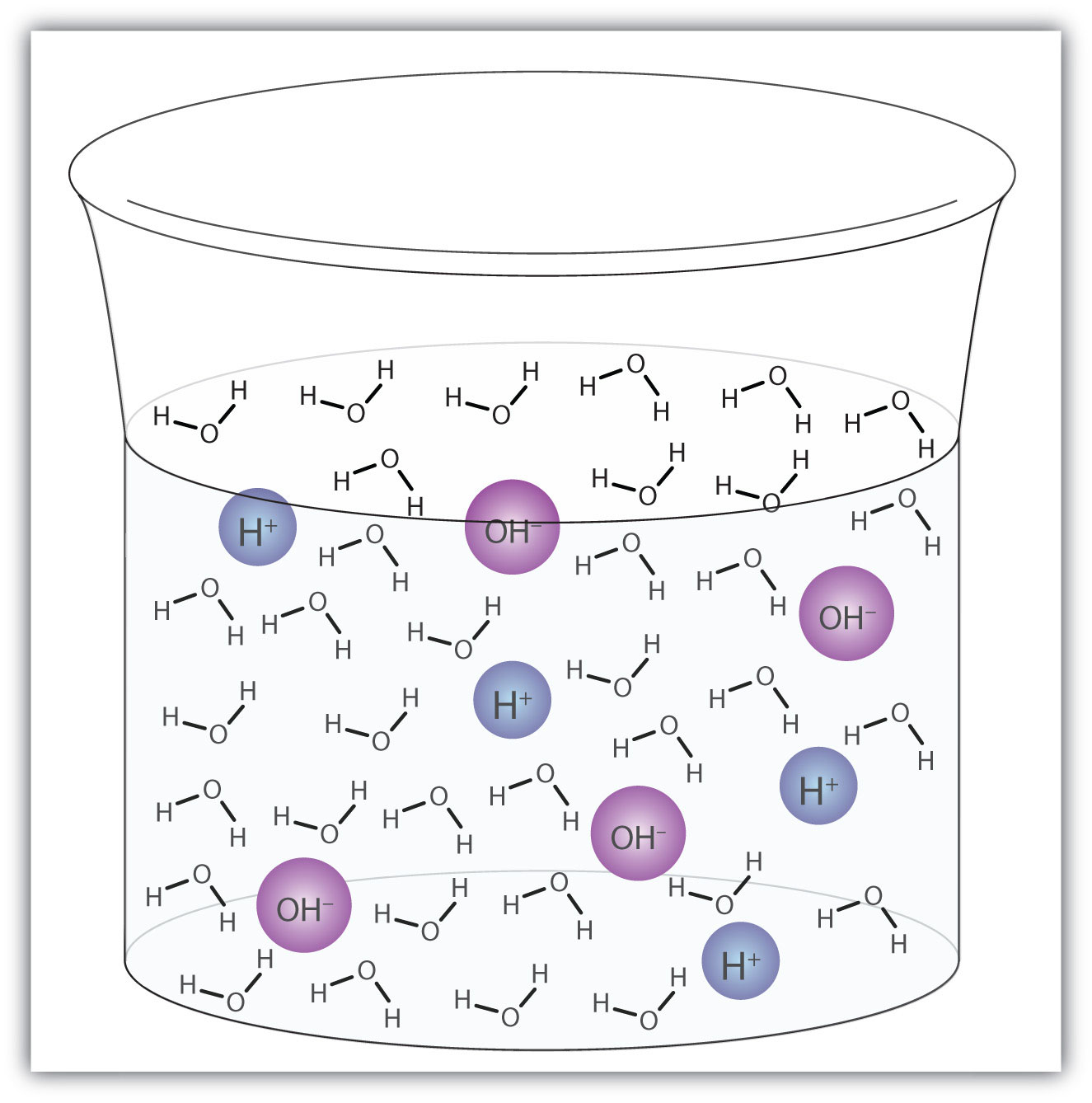## 10.3 Water: Both an Acid and a Base

### Learning Objective

1. Write chemical equations for water acting as an acid and as a base.

Water (H2O) is an interesting compound in many respects. Here, we will consider its ability to behave as an acid or a base.

In some circumstances, a water molecule will accept a proton and thus act as a Brønsted-Lowry base. We saw an example in the dissolving of HCl in H2O:

HCl + H2O(ℓ) → H3O+(aq) + Cl(aq)

In other circumstances, a water molecule can donate a proton and thus act as a Brønsted-Lowry acid. For example, in the presence of the amide ion (see Example 4 in Section 10.2 "Brønsted-Lowry Definition of Acids and Bases"), a water molecule donates a proton, making ammonia as a product:

H2O(ℓ) + NH2(aq) → OH(aq) + NH3(aq)

In this case, NH2 is a Brønsted-Lowry base (the proton acceptor).

So, depending on the circumstances, H2O can act as either a Brønsted-Lowry acid or a Brønsted-Lowry base. Water is not the only substance that can react as an acid in some cases or a base in others, but it is certainly the most common example—and the most important one. A substance that can either donate or accept a proton, depending on the circumstances, is called an amphiproticA substance that can either donate or accept a proton, depending on the circumstances. compound.

A water molecule can act as an acid or a base even in a sample of pure water. About 6 in every 100 million (6 in 108) water molecules undergo the following reaction:

H2O(ℓ) + H2O(ℓ) → H3O+(aq) + OH(aq)

This process is called the autoionization of waterThe process by which water ionizes into hydronium ions and hydroxide ions as it acts as an acid and a base. (Figure 10.2 "Autoionization") and occurs in every sample of water, whether it is pure or part of a solution. Autoionization occurs to some extent in any amphiprotic liquid. (For comparison, liquid ammonia undergoes autoionization as well, but only about 1 molecule in a million billion (1 in 1015) reacts with another ammonia molecule.)

Figure 10.2 AutoionizationA small fraction of water molecules—approximately 6 in 100 million—ionize spontaneously into hydronium ions and hydroxide ions. This picture necessarily overrepresents the amount of autoionization that really occurs in pure water.

### Example 5

Identify water as either a Brønsted-Lowry acid or a Brønsted-Lowry base.

1. H2O(ℓ) + NO2(aq) → HNO2(aq) + OH(aq)
2. HC2H3O2(aq) + H2O(ℓ) → H3O+(aq) + C2H3O2(aq)

Solution

1. In this reaction, the water molecule donates a proton to the NO2 ion, making OH(aq). As the proton donor, H2O acts as a Brønsted-Lowry acid.
2. In this reaction, the water molecule accepts a proton from HC2H3O2, becoming H3O+(aq). As the proton acceptor, H2O is a Brønsted-Lowry base.

### Skill-Building Exercise

Identify water as either a Brønsted-Lowry acid or a Brønsted-Lowry base.

1. HCOOH(aq) + H2O(ℓ) → H3O+(aq) + HCOO(aq)

2. H2O(ℓ) + PO43−(aq) → OH(aq) + HPO42−(aq)

### Concept Review Exercises

1. Explain how water can act as an acid.

2. Explain how water can act as a base.

1. Under the right conditions, H2O can donate a proton, making it a Brønsted-Lowry acid.

2. Under the right conditions, H2O can accept a proton, making it a Brønsted-Lowry base.

### Key Takeaway

• Water molecules can act as both an acid and a base, depending on the conditions.

### Exercises

1. Is H2O(ℓ) acting as an acid or a base?

H2O(ℓ) + NH4+(aq) → H3O+(aq) + NH3(aq)
2. Is H2O(ℓ) acting as an acid or a base?

CH3(aq) + H2O(ℓ) → CH4(aq) + OH(aq)
3. In the aqueous solutions of some salts, one of the ions from the salt can react with water molecules. In some C2H3O2 solutions, the following reaction can occur:

C2H3O2(aq) + H2O(ℓ) → HC2H3O2(aq) + OH(aq)

Is H2O acting as an acid or a base in this reaction?

4. In the aqueous solutions of some salts, one of the ions from the salt can react with water molecules. In some NH4+ solutions, the following reaction can occur:

NH4+(aq) + H2O → NH3(aq) + H3O+(aq)

Is H2O acting as an acid or a base in this reaction?

5. Aluminum hydroxide [Al(OH)3] is amphoteric; it reacts with both acids and bases. Propose the chemical equations for the reactions of Al(OH)3 with H+ and with OH.

6. Based on the information in this section, does ammonia (NH3) autoionize more or less than water? Write the chemical equation for the autoionization of ammonia.